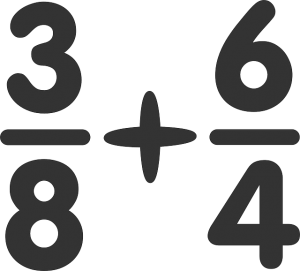## GMAT Fractions and Decimals: Everything You Need to KnowAfter integers, fractions and decimals are usually the next most frequently tested concepts in the GMAT Quant section. The good news is, the math itself is fairly simple: you’ve likely learned all the rules you need to know about working with fractions and decimals in middle and early high school math. The bad news is that these rules and properties have probably been gathering dust in some unvisited corner of your brain—and even if they haven’t, you’re going to have to apply them in new ways on the GMAT.

Never fear! In this post, we’ll tell you everything you need to know about fractions and decimals for the GMAT. We’ll give you a refresher on all the relevant rules and formulas, tips and tricks for every question you’ll see on them on the GMAT, and some example questions with thorough explanations so you can see these strategies in action.
Continue reading “GMAT Fractions and Decimals: Everything You Need to Know”

## GMAT Statistics and Standard Deviation Questions: 4 Key TipsIf Hayley takes the GMAT three times and scores 750, 770, and 800, respectively, what is her mean score? You probably won’t get a statistics question that’s quite that easy on the GMAT, but the good news about GMAT statistics questions is that they test mostly simple concepts. You don’t need to know any complicated formulas or equations to master statistics for GMAT quant questions, but you do need to develop a solid understanding of descriptive statistics principles.

In this article, I’ll give you an overview of what’s tested in GMAT statistics questions, define the four key definitions you need to know, and give you tips for mastering statistics questions. I’ll also walk you through two GMAT statistics sample questions. And, as an added bonus, I’ll offer up a range of terrible statistics puns. (Get it?) By the end of this article, you’ll have mastered statistics for GMAT!
Continue reading “GMAT Statistics and Standard Deviation Questions: 4 Key Tips”

## GMAT Percentages: 5 Key Tips for Percent ProblemsPercents are one of the most common math concepts: in fact, you probably use them every day, whether calculating a tip or determining how much a discount really is. It’s not surprising, then, that GMAT percentage questions pop up throughout the quant section. You may see percents tested by themselves, or they might be part of another question type, such as a geometry question.

Unfortunately, GMAT percent problems can be quite tricky even though the concepts they test are fairly simple. But don’t worry! In this guide, I’ll explain exactly what you need to know to master percentage questions on the GMAT and walk you through sample problems using percents.

## Every GMAT Geometry Formula You Need to KnowIf you’re like me, you probably spent a lot of time in high school memorizing the difference between sine and cosine and sighing over long, multi-step proofs, only to forget all of this hard-earned knowledge the second that classes dismissed for break.

If you’ve forgotten a lot of your high school geometry rules or are just in need of a refresher before taking the GMAT, then you’ve found the right article. In this article, I’ll be giving you a comprehensive overview of GMAT geometry.

First, I’ll talk about what and how much geometry is actually on the GMAT. Next, I’ll give you an overview of the most important GMAT geometry formulas and rules you need to know. Then, I’ll show you four geometry sample questions and explain how to solve them. Finally, I’ll talk about how to study for the geometry you’ll encounter on the GMAT and give you tips for acing test day.

## The Ultimate GMAT Guide to Integer PropertiesIntegers are one of the key recurring elements on the GMAT Quant section, so if you’ve started studying, you probably have some questions. What is an integer? Is zero an integer? What does it mean if integers are consecutive?

The good news is, you’ve almost certainly learned everything you need to know about working with integers in middle and early high school math. The bad news is that these rules and properties have probably been relegated to dusty, moss-ridden corner of your brain—and even if they haven’t, you’re going to have to apply them in novel ways on the exam.

Luckily, you’ve come to the right place! In this post, we’ll tell you everything you need to know about integers for the GMAT. We’ll give you a refresher on all the relevant rules and properties of integers, tips and tricks for every kind of integer question you’ll see on the GMAT, and some example questions with thorough explanations so you can see these strategies in action.

## 4 Key Rules for GMAT Probability QuestionsIf Hayley studies for five hours a day for 30 days leading up to the GMAT, what’s the probability that she’ll meet her goal GMAT score?

While you won’t see a question exactly like that on the GMAT, you’ll encounter a number of probability questions on the quantitative section of the GMAT. Many GMAT test-takers are intimidated by probability questions, but if that’s you, don’t worry! In this guide, I’ll teach you everything you need to know to conquer probability on the GMAT.

First, I’ll walk you through the four essential GMAT probability rules you’ll need to answer every probability question. Then, I’ll offer several other tips for acing probability questions. Next, I’ll walk you through four sample GMAT probability questions with explanations. Finally, I’ll give you some tips on practicing for probability questions. What’s the probability that you’ll ace  probability GMAT questions after reading this guide? Pretty high, I’d say.